# MA2051 - Ordinary Differential EquationsSolutions to Second Sample Exam Originally Given 1996 D Term

1.
(a) Just integrate: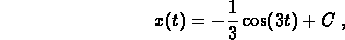and use the initial data: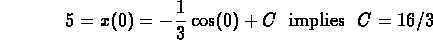(b)
Separate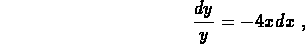integrate and simplify to obtainUse the initial data to find C: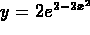2. You are solving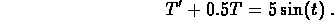(a)
The corresponding homogeneous equation is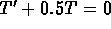. The characteristic equation is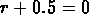, and soand the homogeneous solution is.
(b)
Use the method of Undetermined Coefficients and look for a solution of the form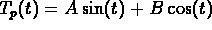. Plug this into the original equation and equate coefficients to obtain A = 2 and B = -4.
(c)
The general solution is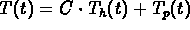. The initial condition gives you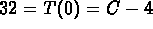and so C = 36.
(d)
The answer is yes: simply choose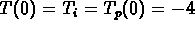. (You just have to make the initial data match the initial value for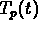.)

3. Let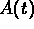denote the amountof carbon monoxide in the lecture hall at time t (in cubic meters) and let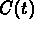denote the concentrationof carbon monoxide in the hall at time t (in cubic meters per cubic meter). Then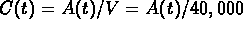.

(a)
CO enters the hall at rate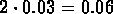(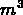/minute).

(b)
CO leaves the hall at the rate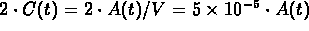(/minute).

(c)
The balance equation is Net change in the amount between t and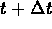= (rate in)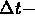(rate out)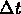,

or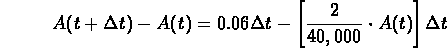(d)
Collect terms, divide byand take the limit asapproaches zero to obtain the model:To turn this into an equation for the concentration, replacebyeverywhere that you see it.

4. Sketch the graph of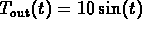. The differential equation tells you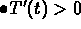when (or where)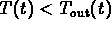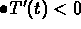when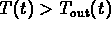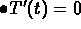when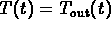The last condition tells you that the inside temperature can have a max or a min only when the the solution curve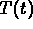crosses the graph of. It also tells you thatcannot follow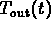exactly (because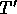would have to be zero everywhere along the solution).

For the initial data givenstarts at 0 and stays strictly belowuntil the curves cross (at some time after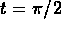when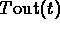is strictly less than 10). This shows that the amplitude of th inside temperature is smaller than the amplitude of the outside temperature.

```

© 1996 by MA2051 Webmasters

All rights Reserved. File last modified on

```